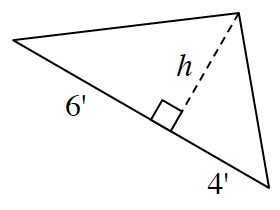### Home > INT2 > Chapter 2 > Lesson 2.2.1 > Problem2-53

2-53.

The area of the triangle at right is $25$ square feet. Determine the value of $h$. Then calculate the perimeter of the entire triangle. Show all work.

Use the formula for the area of a triangle to find the height.

$25 = (0.5)(6 + 4)(h)$